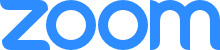# Omsk Algebraic Webinar

Upcoming meeting:

### "Description of the coordinate groups of irreducible algebraic sets over free $$2$$-nilpotent groups"

Abstract. The talk is based on a joint work with M. Amaglobeli (Tbilisi State university) and V. Remeslennikov (Sobolev Institute of Mathematics).

We give a pure algebraic description of the coordinate groups of irreducible algebraic sets over non-abelian free $$2$$-nilpotent group $$N$$. As a corollary we describe finitely generated groups $$H$$ which are universally equivalent to the group $$N$$ (with constants from $$N$$ in the language). Besides, we give a pure algebraic criterion when a group $$H$$, containing $$N$$ as a subgroup, and $$N$$-separated by $$N$$, is in fact $$N$$-discriminated by $$N$$.The meeting will be held using the Zoom platform. Join us via browser Or join us with Zoom Id: 812 2079 3393

Latest announcements:

### "Geometrical Equivalence and Action Type Geometrical Equivalence of Group Representations"

Abstract. The universal algebraic geometry of group representations was considered in: B. Plotkin, A. Tsurkov, “Action type geometrical equivalence of group representations”, Algebra and discrete mathematics, 4: (2005), 48-79. The concepts of geometrical equivalence and action type geometrical equivalence of group representations were defined. It was proved that if two representations are geometrically equivalent then they are action type geometrically equivalent. Also it was remarked that if two representations $$(V_1,G_1)$$ and $$(V_2,G_2)$$ are action type geometrically equivalent and groups G₁ and G₂ are geometrically equivalent, the representations $$(V_1,G_1)$$ and $$(V_2,G_2)$$ are not necessarily geometrically equivalent. However some specific counterexample was not presented. In this talk we fill the gap and construct the example of two representations $$(V_1,G_1)$$ and $$(V_2,G_2)$$ which are action type geometrically equivalent and groups $$G_1$$ and $$G_2$$ are geometrically equivalent, but the representations $$(V_1,G_1)$$ and $$(V_2,G_2)$$ are not geometrically equivalent.

### "Plotkin`s problem for semigroups"

Abstract. B.Plotkin posed the following problem: when a wreath product of two groups is $$q_{\omega}$$-compact or equationally Noetherian? We solve this problem for wreath products of semigroups $$A$$ wr $$B$$ where $$B$$ is infinite cyclic and $$A$$ contains zero.

### "Automorphisms of the category of free algebras"

Abstract. There are many various reasons why it is interesting to study automorphisms of some arbitrary algebraic structure. Our considerations are tightly connected with some problems in Universal Algebraic Geometry. The main goal of this talk is give a brief introduction to Universal Algebraic Geometry and to describe the method of verbal operations for a description of automorphisms of the category free finitely generated algebras in a given variety of algebras.

### "Sublinear time algorithms in (semi)groups"

Abstract. We are going to discuss what can be done in sublinear time (in the "length" of an input); in particular, without reading the whole input but only a small part thereof. One well-known example is deciding divisibility of a decimal integer by $$2$$, $$5$$, or $$10$$: this is done by reading just the last digit. We will discuss some less obvious examples from (semi)group theory.

### "IBN-varieties of algebras"

Abstract. The concept of a variety with IBN (invariant basic number) property first appeared in the ring theory. But we can define this concept for an arbitrary variety of universal algebras with an arbitrary signature. The proof of the IBN propriety of some variety is very important in universal algebraic geometry. This is a milestone in the study of the relation between geometric and automorphic equivalences of algebras of this variety. We prove very simple but very useful for the study of IBN properties of different varieties theorems. We will consider some applications of these theorems. We will consider many-sorted universal algebras as well as one-sorted. So, all concepts and all results will be generalized for the many-sorted case.

### "Equationally noetherian graphs and hypergraphs"

Abstract. A simple graph is called equationally noetherian if any system of equations is equivalent to its finite subsystem. It isn't hard to notice that a simple graph is equationally noetherian if and only if it is equationally noetherian in one variable equations. Based on this property we described all equationally noetherian graphs with help of forbidden subgraphs (joint with Ivan Buchinsky). Also several examples of hypergraphs will be presented that are not equationally noetherian but equationally noetherian in one variable equations.

### "Hardness of equations over finite solvable groups under the exponential time hypothesis"

Abstract. The study of the complexity of the equation satisfiability problem in finite groups had been initiated by Goldmann and Russell in 2002. They showed that this problem is in polynomial time for nilpotent groups, while it is $$NP$$-complete for non-solvable groups. Since then, several results have appeared showing that the problem can be solved in polynomial time in certain solvable groups G having a nilpotent normal subgroup H with nilpotent factor $$G/H$$. In this talk I will show that such normal subgroup must exist in each finite group with equation satisfiability solvable in polynomial time, unless the Exponential Time Hypothesis fails. Moreover, the same hardness result applies to the equation identity problem. The talk is based on joint work with Pawel Idziak, Piotr Kawalek, and Jacek Krzaczkowski.